# NCERT Solutions class 7 Maths Chapter-5 Exercise 5.1

NCERT Solutions Class 7 Maths chapter-5 line and angles Exercise-5.1 is prepared by academic team of Physics Wallah all the questions of NCERT text book are solved step by step with proper and detail solutions explaining each and every questions. For More and additional questions of CBSE class 7 maths you can go to class 7 maths sections. NCERT class 7 Maths Solutions is the best way to enhanced your mathematics skill. And Physics Wallah practice worksheet & question bank will help you a lot.

## line and angles Exercise-5.1

### Solutions of Chapter line and angles Exercise-5.1

Question 1:
Find the complement of each of the following angles: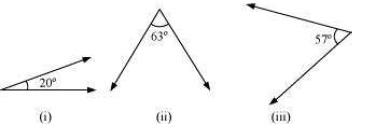The sum of the measures of complementary angles is 90º.
(i) 20°
Complement = 90° − 20°
= 70°
(ii) 63°
Complement = 90° − 63°
= 27°
(iii) 57°
Complement = 90° − 57°
= 33°

Question 2:
Find the supplement of each of the following angles: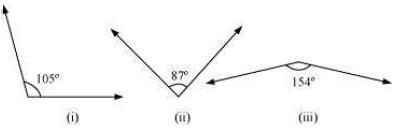The sum of the measures of supplementary anglesis 180º.
(i) 105°
Supplement = 180° − 105°
= 75°
(ii) 87°
Supplement = 180° − 87°
= 93°
(iii) 154°
Supplement = 180° − 154°
= 26°

Question 3:
Identify which of the following pairs of angles are complementary and which are supplementary.
(i) 65°, 115° (ii) 63°, 27°
(iii) 112°, 68° (iv) 130°, 50°
(v) 45°, 45° (vi) 80°, 10°
The sum of the measures of complementary angles is 90º and that of supplementary anglesis 180º.
(i) 65°, 115°
Sum of the measures of these angles = 65º + 115º = 180°
∴ These angles are supplementary angles.
(ii) 63°, 27°
Sum of the measures of these angles = 63º + 27º = 90°
∴ These angles are complementary angles.
(iii) 112°, 68°
Sum of the measures of these angles = 112º + 68º = 180°
∴ These angles are supplementary angles.
(iv) 130°, 50°
Sum of the measures of these angles = 130º + 50º = 180°
∴ These angles are supplementary angles.
(v) 45°, 45°
Sum of the measures of these angles = 45º + 45º = 90°
∴ These angles are complementary angles.
(vi) 80°, 10°
Sum of the measures of these angles = 80º + 10º = 90°
∴ These angles are complementary angles.

Question 4:
Find the angle which is equal to its complement.
Let the angle be x.
Complement of this angle is also x.
The sum of the measures of a complementary angle pair is 90º.
∴ x + x = 90°
2x = 90°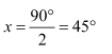Question 5:
Find the angle which is equal to its supplement.
Let the angle be x.
Supplement of this angle is also x.
The sum of the measures of a supplementary angle pair is 180º.
∴ x + x = 180°
2x = 180°
x = 90°

Question 6:
In the given figure, ∠1 and ∠2 are supplementary angles. If ∠1 is decreased, what changes should take place in ∠2 so that both the angles still remain supplementary.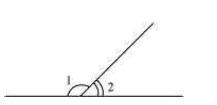∠1 and ∠2 are supplementary angles.
If ∠1 is reduced, then ∠2 should be increased by the same measure so that this angle
pair remains supplementary.

Question 7:
Can two angles be supplementary if both of them are:
(i) Acute? (ii) Obtuse? (iii) Right?
(i) No. Acute angle is always lesser than 90º. It can be observed that two angles, even of 89º, cannot add up to 180º. Therefore, two acute angles cannot be in a supplementary angle pair.
(ii) No. Obtuse angle is always greater than 90º. It can be observed that two angles, even of 91º, will always add up to more than 180º. Therefore, two obtuse angles cannot be in a supplementary angle pair.
(iii) Yes. Right angles are of 90º and 90º + 90º = 180°
Therefore, two right angles form a supplementary angle pair together.

Question 8:
An angle is greater than 45°. Is its complementary angle greater than 45° or equal to 45° or less than 45°?
Let A and B are two angles making a complementary angle pair and A is greater than 45º.
A + B = 90º
B = 90º − A
Therefore, B will be lesser than 45º.

Question 9:
(i) Is ∠1 adjacent to ∠2?
(ii) Is ∠AOC adjacent to ∠AOE?
(iii) Do ∠COE and ∠EOD form a linear pair?
(iv) Are ∠BOD and ∠DOA supplementary?
(v) Is ∠1 vertically opposite to ∠4?
(vi) What is the vertically opposite angle of ∠5?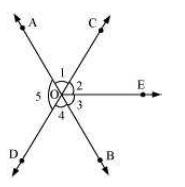(i) Yes. Since they have a common vertex O and also a common arm OC. Also, their non-common arms, OA and OE, are on either side of the common arm.
(ii) No. They have a common vertex O and also a common arm OA. However, their noncommon arms, OC and OE, are on the same side of the common arm. Therefore, these are not adjacent to each other.
(iii) Yes. Since they have a common vertex O and a common arm OE. Also, their noncommon arms, OC and OD, are opposite rays.
(iv) Yes. Since ∠BOD and ∠DOA have a common vertex O and their non-common arms are opposite to each other.
(v) Yes. Since these are formed due to the intersection of two straight lines (AB and CD).
(vi) ∠COB is the vertically opposite angle of ∠5 as these are formed due to the intersection of two straight lines, AB and CD.

Question 10:
Indicate which pairs of angles are:
(i) Vertically opposite angles. (ii) Linear pairs.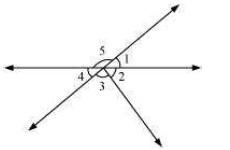(i) ∠1 and ∠4, ∠5 and ∠2 +∠3 are vertically opposite angles as these are formed due to the intersection of two straight lines.
(ii) ∠1 and ∠5, ∠5 and ∠4 as these have a common vertex and also have non-common arms opposite to each other.

Question 11:
In the following figure, is ∠1 adjacent to ∠2? Give reasons.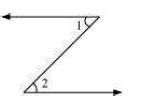∠1 and ∠2 are not adjacent angles because their vertex is not common.

Question 12:
Find the value of the angles x, y, and z in each of the following: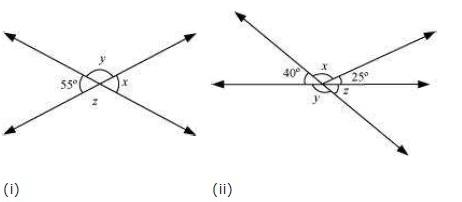(i) Since ∠x and ∠55° are vertically opposite angles,
∠x = 55°
∠x + ∠y = 180° (Linear pair)
55° + ∠y = 180°
∠y = 180º − 55º = 125°
∠y = ∠z (Vertically opposite angles)
∠z = 125°
(ii) ∠z = 40° (Vertically opposite angles)
∠y + ∠z = 180° (Linear pair)
∠y = 180° − 40° = 140°
40° + ∠x + 25° = 180° (Angles on a straight line)
65° + ∠x = 180°
∠x = 180° − 65° = 115°

Question 13:
Fill in the blanks:
(i) If two angles are complementary, then the sum of their measures is _______.
(ii) If two angles are supplementary, then the sum of their measures is _______.
(iii) Two angles forming a linear pair are _______.
(iv) If two adjacent angles are supplementary, they form a _______.
(v) If two lines intersect at a point, then the vertically opposite angles are always _______.
(vi) If two lines intersect at a point, and if one pair of vertically opposite angles are acute angles, then the other pair of vertically opposite angles are _______.
(i) 90°
(ii) 180°
(iii) Supplementary
(iv) Linear pair
(v) Equal
(vi) Obtuse angles

Question 14:
In the adjoining figure, name the following pairs of angles.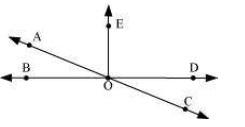(i) Obtuse vertically opposite angles
(iii) Equal supplementary angles
(iv) Unequal supplementary angles
(v) Adjacent angles that do not form a linear pair